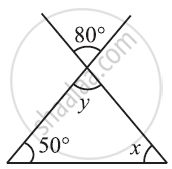Share

# Find the Value of the Unknowns X and Y in the Following Diagrams: - 2 - CBSE Class 7 - Mathematics

ConceptAngle Sum Property of a Triangle

#### Question

Find the value of the unknowns x and y in the following diagrams:#### Solution

y = 80° (Vertically opposite angles)

y + x + 50° = 180° (Angle sum property)

80° + x + 50° = 180°

x + 130º = 180°

x = 180° − 130º = 50°

Is there an error in this question or solution?

#### APPEARS IN

NCERT Solution for Mathematics for Class 7 (2018 to Current)
Chapter 6: The Triangle and its Properties
Ex. 6.30 | Q: 2.2 | Page no. 121
Solution Find the Value of the Unknowns X and Y in the Following Diagrams: - 2 Concept: Angle Sum Property of a Triangle.
S